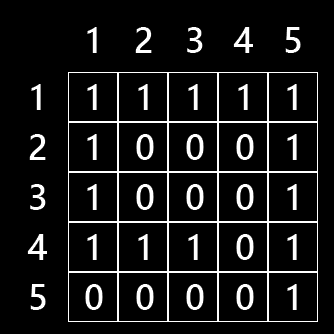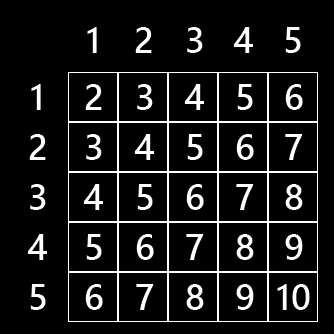# How to draw an isometric grid

## How to draw isometric

I got asked on Reddit how I did the isometric rendering for Hallways, so I'll try to explain. Disclaimer: I learned coding by trying stuff until something works. Chances are my methods are very inefficient or just plain wrong.

### Step 1: Create a level

For this example, we'll just have a 5x5 grid where ones represent walls and zeroes represent floors.The top left corner (coordinate 1,1) will eventually be drawn in the center back.

### Step 2: Sort by depth

Because the top left corner will be in the back, we want that one to be drawn first, followed by square (2,1) and (1,2). After that we need to draw (1,3), (2,2) and (3,1), and so forth.

The difficult part to me was figuring out a simple formula to sort squares by depth. Until I took a closer look at those coordinates. Turned out we can just add up the x and y coordinates to get the depth number. In my game, I called this number the index. Here's the indices for all cells in our tiny level:For calculation purposes, it's easiest to then subtract 2 from every index so the backmost cell has an index of zero.

### Step 3: Draw from back to front

Now we just need to cycle through all cells from lowest to highest index and draw them. To make sure back cells end up on top of the screen and front cells on the bottom, we get the y coordinate by multiplying the index by half the height of a cell. To get the x, subtract the original y of the cell from the original x and multiply the result by half the width of a cell.

Remember to draw the cells as diamonds (by using a polygon, two triangles or a diamond-shaped sprite) instead of squares, otherwise it will just look weird.

To draw objects or actors in the level, we can cycle through all objects and actors every time we draw a cell and draw the ones with matching coordinates. This might get really slow and unresponsive on bigger levels with lots of content, but it worked on my project.

### Step 4: Profit!

I hope this explanation is clear and is helpful for someone. If you need more help or more detailed instructions, just let me know.

## Files

Hallways 1,014 kB
Oct 22, 2019